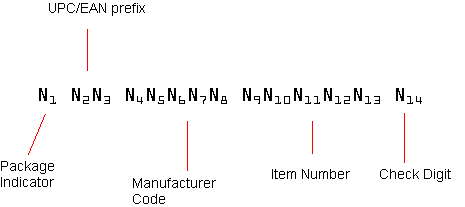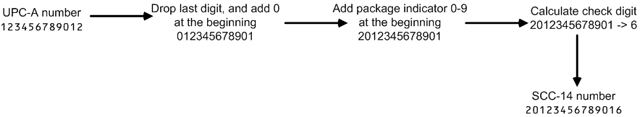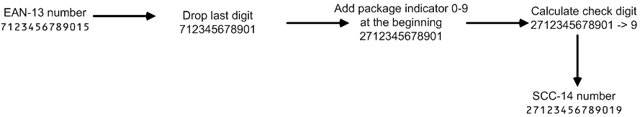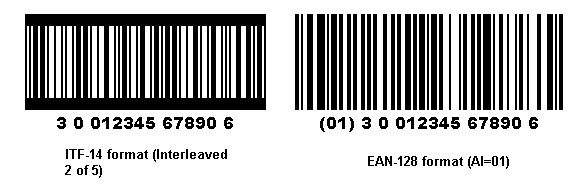Search For Category All ArticlesBarcode Fonts    General    Crystal Reports    PCL Fonts    Reporting Service    ProgrammingBarcode ComponentsLabeling SoftwareBarcode Knowledge    Symbology SpecificationsMorovia Web Site

## Shipping Container Code (GS1-14, SCC-14, GTIN-14)

The GS1-14, EAN/UCC-14, also called SCC-14, GTIN, DUN-14 or U.P.C. Shipping Container Code, is a 14-digit number assigned to fixed-content shipping containers. SCC-14 can have two types of barcode representations. The most widely used representation is UCC/EAN-128 format, with Application Identifier (AI) 01. The ITF-14, which is based on Interleaved 2 of 5, is also frequently used.

A SCC-14 number consists of 14 digits that begin with an indicator digit and end with a check digit. The check digit is calculated according to the UCC/EAN algorithm (same as UPC/EAN check digit). Note that this check digit is usually not the same as the check digit in a UPC-A or EAN-13 number, unless the package indicator is 0.

Figure 1. SCC-14 number structureA SCC-14 number contains the following information:

• Digit 1: Package indicator (PI)

• Digits 2-3: UPC numbering system/EAN country prefix. (GS1 country prefix)

• Digits 4-8: GS1 Company Prefix (can be longer)

• Digits 9-13: Item identification number

• Digit 14: Check digit

The first digit, Package Indicator (PI), indicates package variant. For example, you package the same product into two different cases with one case containing 10 items and the another containing 12. You assign 1 for the first case and 2 for the second. However, there is no standard mandating that how many items should be placed in the case for PI=1. The quantity is checked against the database.

In two cases you may assign PI=0. One case is to indicate that the item number identifying the container is different from the item number on the units inside the container. The other case is when the container contains one unit of the item. When stored in the database, a 12-digit UPC or 13-digit EAN code are stored into a 14-digit numeric format. The 14-digit is identical to the SCC-14 with PI=0.

To calculate SCC-14 number from a UPC-A number, first drop the last check digit. Add 0 at the beginning to make a 12-digit number (the digit 0 is the implicit country code for US and Canada). Add the package indicator at the beginning to make up 13 digits. Calculate the check digit based on this 13-digit number (See section below) and append it to the end. The result is SCC-14.

Figure 2. Converting UPC-A number to SCC-14To derive the SCC-14 number from an EAN-13 number, first drop the last check digit. Add the package indicator at the beginning and calculate the SC-14 checksum. Append the new check digit to the end and you get the SCC-14 number.

Figure 3. Converting EAN-13 number to SCC-14## SCC-14 Barcode Format

A SCC-14 number is usually encoded with Code128 symbology (GS1-128). Since GS1-128 is also used to encode other information in the shipping label, two digits, called Application Identifier (AI) are added at the beginning of the barcode. The AI for SCC-14 is 01.

Figure 4. SCC-14 BarcodesSCC-14 can also be depicted in Interleaved 2 of 5 format. In the images above, the left one is Interleaved 2 of 5 format, and the right one is drawn in GS1-128. Both images were created with Monterey Barcode Creator.

Update: since we published this article, we have received many inquiries on how to determine the barcode format. A simple method is to count the number of bars. If the barcode is in interleaved 2 of 5 format, it should have 39 bars in total. For barcodes in EAN-128 format, the number is 37. Another way is to examine the end of the barcode. The last three bars of an EAN-128 barcode are always in 3x, 1x, and 2x of the smallest width (X dimension).

## Check Digit Calculation

SCC-14 check digit is calculated the same way as calculating checksum for UPC-A/EAN-13 numbers. Below are the steps to calculate SCC-14 check digit:

• From the right to left, start with odd position, assign the odd/even position to each digit.

• Sum all digits in odd position and multiply the result by 3.

• Sum all digits in even position.

• Sum the results of step 3 and step 4.

• Divide the result of step 4 by 10. The check digit is the number which adds the remainder to 10.

A web-based free utility to calculate SSCC check digit can be found here.